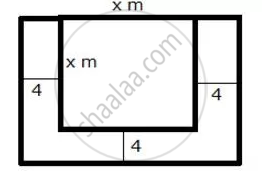Share

A Square Lawn is Bounded on Three Sides by a Path 4 M Wide. If the Area of the Path is 7/8 that of the Lawn, Find the Dimensions of the Lawn. - ICSE Class 10 - Mathematics

Question

A square lawn is bounded on three sides by a path 4 m wide. If the area of the path is 7/8 that of the lawn, find the dimensions of the lawn.

Solution

Let the side of the square lawn be x m.
Area of the square lawn = x2 m2
The square lawn is bounded on three sides by a path which is 4 m wide.Area of outer rectangle = (x + 4) (x + 8) = x2 + 12x + 32
Area of path = x2 + 12x + 32 – x2 = 12x + 32
From the given information, we have:

12x + 32 = 7/8 x^2

96x + 256 = 7x^2

7x^2 - 96x - 256 = 0

7x^2 - 112x + 16x - 256 = 0

7x(x - 16) + 16(x - 16) = 0

(x - 16)(7x + 16) = 0

x = 16, (-16)/7

Since, x cannot be negative. So, x = 16 m.
Thus, each side of the square lawn is 16 m.

Is there an error in this question or solution?

APPEARS IN

Selina Solution for Selina ICSE Concise Mathematics for Class 10 (2018-2019) (2017 to Current)
Chapter 6: Solving (simple) Problems (Based on Quadratic Equations)
Ex.6B | Q: 12

Video TutorialsVIEW ALL 

Solution A Square Lawn is Bounded on Three Sides by a Path 4 M Wide. If the Area of the Path is 7/8 that of the Lawn, Find the Dimensions of the Lawn. Concept: Quadratic Equations.
S